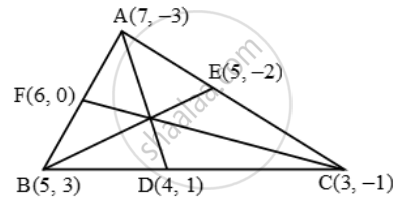# Find the Lengths of the Medians of a ∆ABC Whose Vertices Are A(7, –3), B(5,3) and C(3,–1) - Mathematics

Sum

Find the lengths of the medians of a ∆ABC whose vertices are A(7, –3), B(5,3) and C(3,–1)

#### Solution 1

Let D, E, F be the mid-points of the sides BC, CA and AB respectively. Then, the coordinates of D, E and F are

D( \frac{5+3}{2},\ \frac{3-1}{2})=D(4,\text{ 1}),

E( \frac{3+7}{2},\ \frac{-1-3}{2})=E( 5,-2 )

F((7+5)/2,(3-(-3))/2)=F(6,3)\therefore AD=sqrt((7-4)^{2}+(-3-1)^{2})=\sqrt{9+16}=5

BE=sqrt((5-5)^{2}+(-2-3)^{2})=sqrt{0+25}=5

CF=sqrt((6-3)^{2}+(3-(-1))^{2})=sqrt{9+16}=5

the lengths of the medians is 5

#### Solution 2

The given vertices are A(7, –3), B(5,3) and C(3,–1).

Since D and E are the midpoints of BC and AC respectively. therefore

"Coordinates of " D = ((5+3)/2 , (3-1)/2) = (4,1)

" Coordinates of " E = ((7+3)/2 , (-3-1)/2) = (5,-2)

Now ,

AD = sqrt((7-4)^2 +(-3-1)^2) = sqrt(9+16)=5

BE = sqrt((5-5)^2 +(3+2)^2) = sqrt(0+25) = 5

Hence, AD = BE = 5 units.

Concept: Section Formula
Is there an error in this question or solution?

#### APPEARS IN

RS Aggarwal Secondary School Class 10 Maths
Chapter 16 Coordinate Geomentry
Q 7